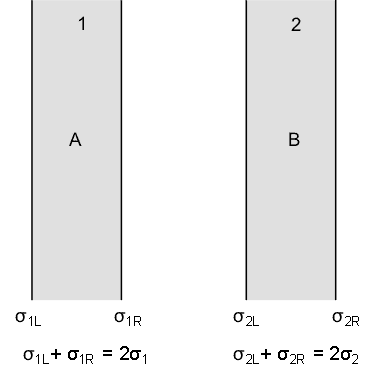# E between parallel plates

wumple
I'm not sure if this qualifies as a 'homework question'. There is no specific problem...I have a question about something in the text.

It gives the situation of a conducting plate with charge density sigma 1 on each side. The E field on each side due to the conducting plate is = sigma 1/epsilon.

When a second conducting plate (plate 2) is brought near, the charge on the opposite sides of each of the plates is attracted to the interior side. So now one side of each plate has charge density 0 and one side has charge density 2 * sigma 1 (or sigma 2).

Then it says that the E field between the plates is equal to 2 *sigma 1/ epsilon or twice what it was before the second plate was brought over.

But why wouldn't the second plate generate a field with equal magnitude, making the total E field in between the plates = 4 * sigma 1 / epsilon? Why is the total E field in between the two plates only calculated from one plate, and not considering both?

Then this website: http://www.ac.wwu.edu/~vawter/PhysicsNet/Topics/Capacitors/ParallCap.html [Broken]

Says that the E fields are equal to (sigma 1)/2 epsilon and that the E fields add. So the book says that the charge densities double but the E fields don't add, and this website says that the charge densities stay the same but the E fields add.

Also, the E field is 0 on the outside of the plates. The website says it is because the E fields cancel. The book says it is because there is no excess charge on the outside of the plates since it all went to the inside. but why the E field be zero just because there is no charge near it? There's still charge on the inside of the plate, just a little farther away.

What's really going on? I'm confused! Help!

Last edited by a moderator:

Homework Helper
Gold Member
2021 Award
Consider a single conducting infinite sheet with surface charge density ##\sigma_1## on each face and total charge density ##2\sigma_1##. The electric field it generates is obtained from Gauss's law. The electric flux through the usual "pillbox" is ##\Phi_E=2EA## and the enclosed charge is ##q_{enc}=2\sigma_1A##. Application of Gauss's law gives the magnitude of the electric field, ##E=\sigma_1/\epsilon_0##. The direction of the field is perpendicular to the sheet and away from it on either side. (we assume that ##\sigma_1>0.##) We can view this as the superposition field produced by two sheets, each generating field ##E=\dfrac{\sigma_1}{2\epsilon_0}.##

So to model this, we charge two sheets, bring them close to each other and let the surface charges go wherever they want. We will consider four surfaces each producing its own field. Using subscripts 1 and 2 to identify the sheets and L and R for "left" and "right", we have $$\sigma_{1L}+\sigma_{1R}=2\sigma_1~~~~~(1);~~\sigma_{2L}+\sigma_{2R}=2\sigma_2~~~~~(2)$$Now look at the drawing below. We know that inside conductor 1 at point A the electric field must be zero. By superposition, it is also the sum of four contributions from the four distributions, one to the right from ##\sigma_{1L}## and the other three to the left.We write for the total field at A, $$\frac{\sigma_{1L}}{2\epsilon_0}-\frac{\sigma_{1R}}{2\epsilon_0}-\frac{\sigma_{2L}}{2\epsilon_0}-\frac{\sigma_{2R}}{2\epsilon_0}=0$$Using equations (1) and (2) and simplifying,$$\sigma_{1L}-(2\sigma_1-\sigma_{1L})-2\sigma_2=0~\rightarrow~\sigma_{1L}=\sigma_1+\sigma_2$$Then$$\sigma_{1R}=2\sigma_1-\sigma_{1L}=\sigma_1-\sigma_2.$$ You can follow the same procedure to find the remaining two distributions by demanding that the electric field be zero at point B or you can note that there is left-to-right symmetry which means that you can swap subscripts "1" and "2" and subscripts "L" and "R" to get,
$$\sigma_{2R}=\sigma_2+\sigma_1~;~~~~~\sigma_{2L}=\sigma_2-\sigma_1.$$Once you have the four distributions, you can find the electric field anywhere. For example, between the plates,$$E_{between}=\frac{1}{2\epsilon_0}(\sigma_{1L}+\sigma_{1R}-\sigma_{2L}-\sigma_{2R})=\frac{\sigma_1-\sigma_2}{\epsilon_0}$$This method is straightforward and can be easily extended to more than two plates. If one of the distributions is given to you as negative, then just flip its sign in the above equations.

On edit 10/12/2020
Crossed out misleading statement in first sentence. The charge density is the same on each face; the total charge is ##2\sigma_1A##.

Last edited: